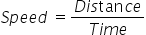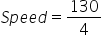Mathematics
Easy

Question

# A skydiver falls 130 feet in 4 seconds. Determine the distance he falls in 9 seconds

## 250.2300.2312292.5Hint:

## The correct answer is: 292.5

### The given values of distance and time are as follows:Distance = 130 feetTime = 4 secondsWe have to find the speed at which the skydiver falls. As the given speed is constant, we can use this distance travelled in 9 seconds.We know the formula for speed.Substituting the values, we get= 32.5 feet per sec.Now, as the rate or speed is constant, we can use this value to find the value of the distance the skydiver falls for any time interval.We have to find the distance travelled in 9 seconds.Let’s see the formulaRearranging the formula to find distance. We will multiply both sides by time.Distance = Speed × Time= 32.5 × 9 seconds= 292.5Therefore, the distance a falls is 292.5 feet.So the option which is ‘292.5’, is the right option.

We have to be careful about the units. Same quantities must have same unit.

### Related Questions to study#### With Turito Foundation.#### Get an Expert Advice From Turito.